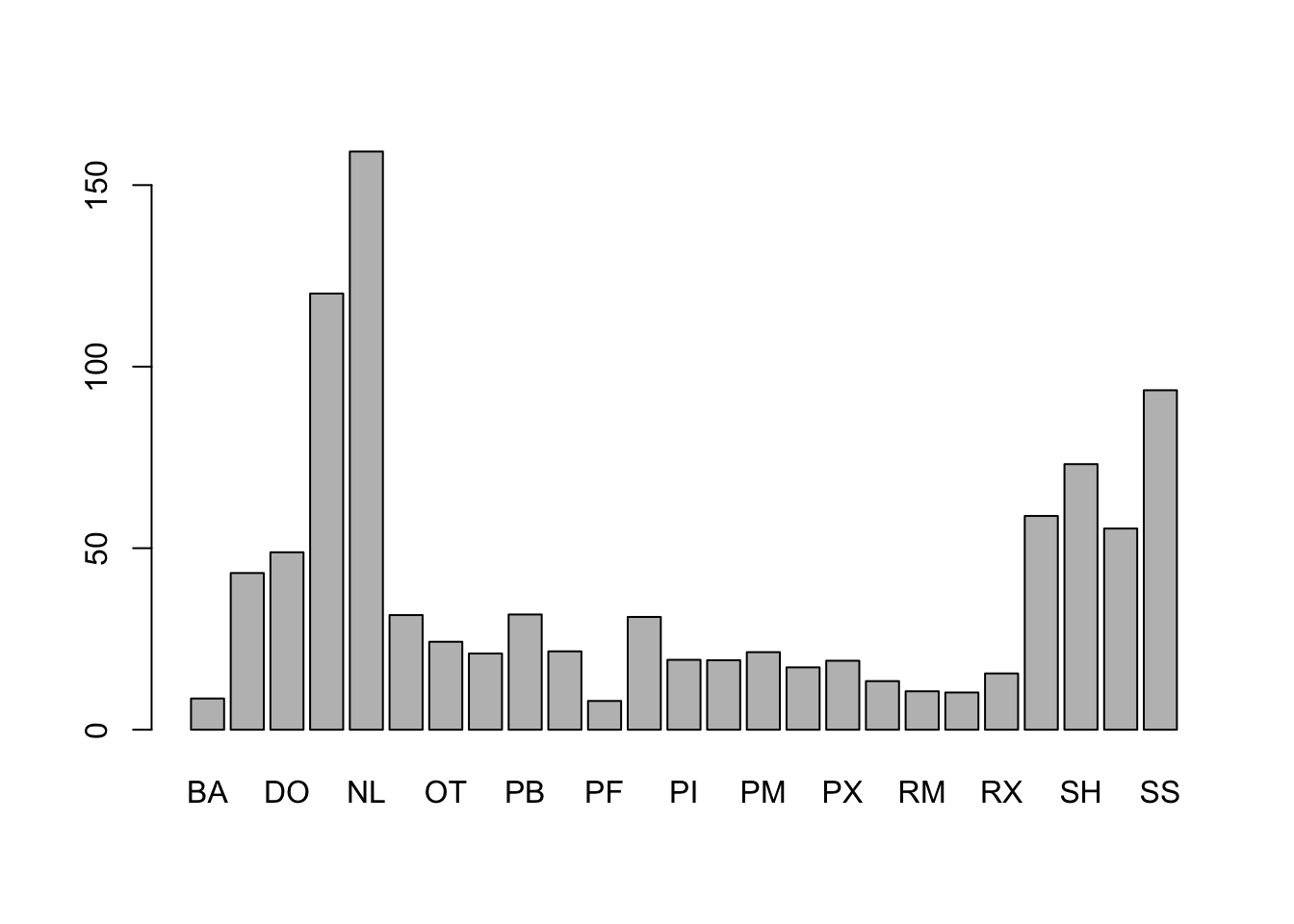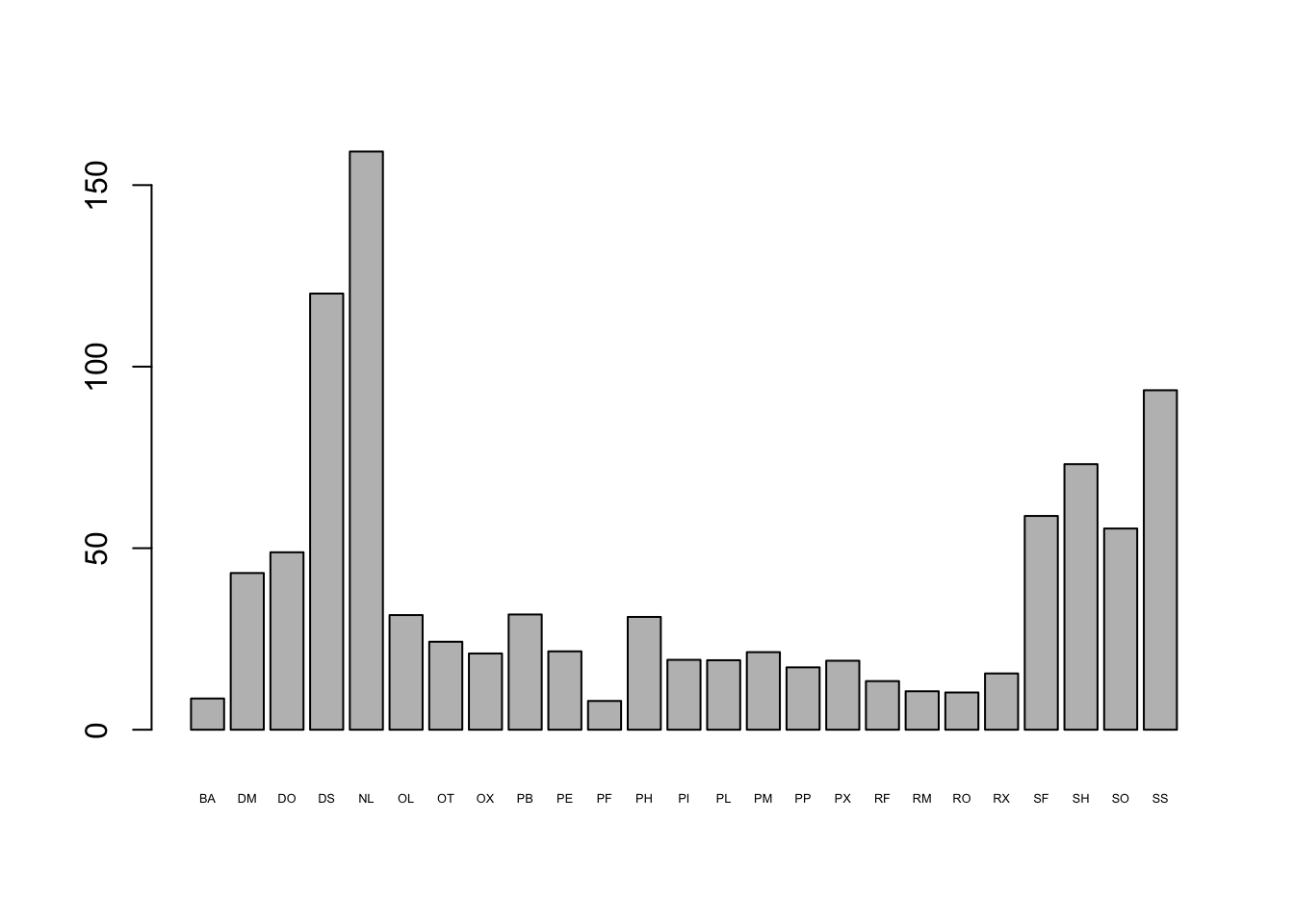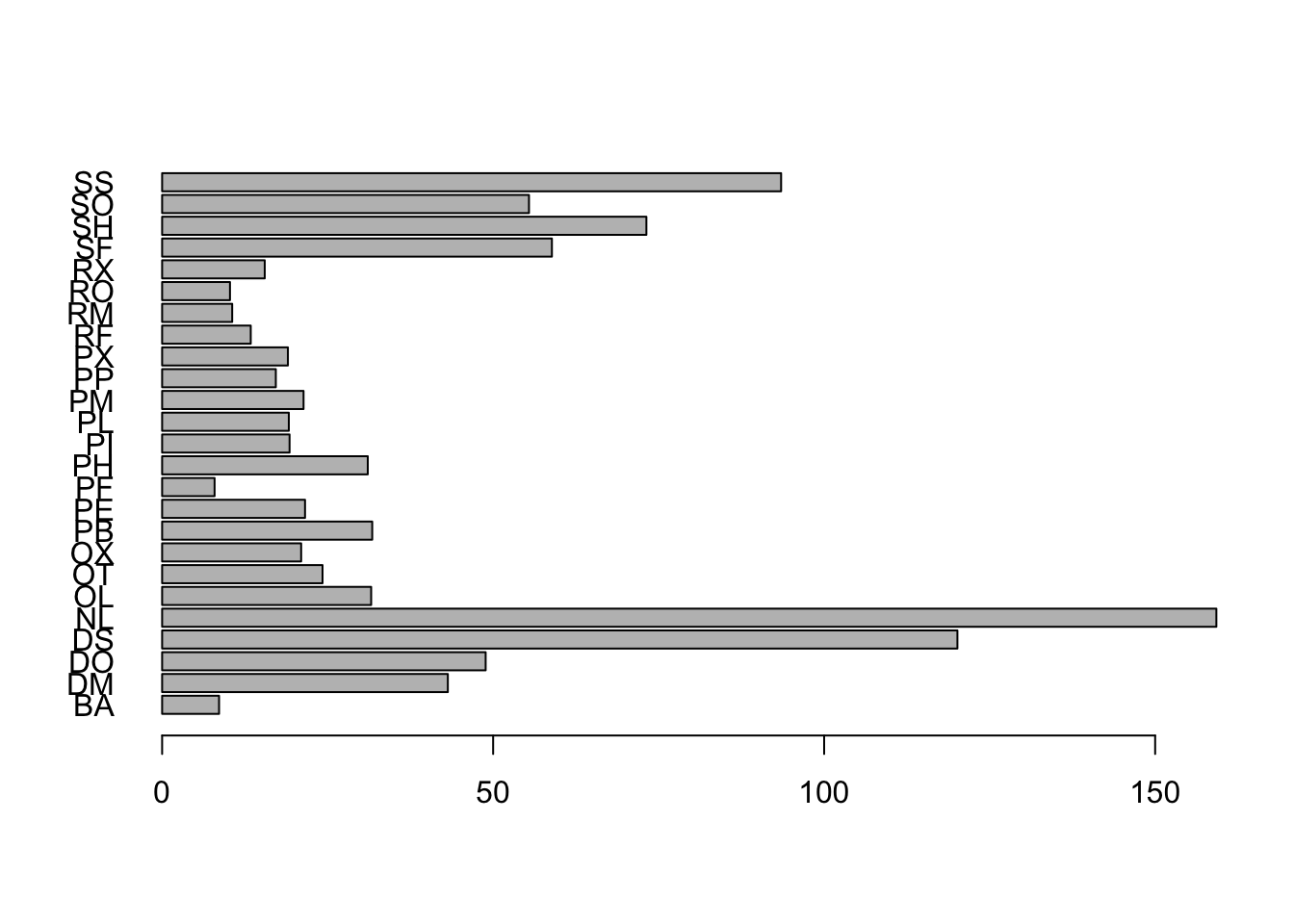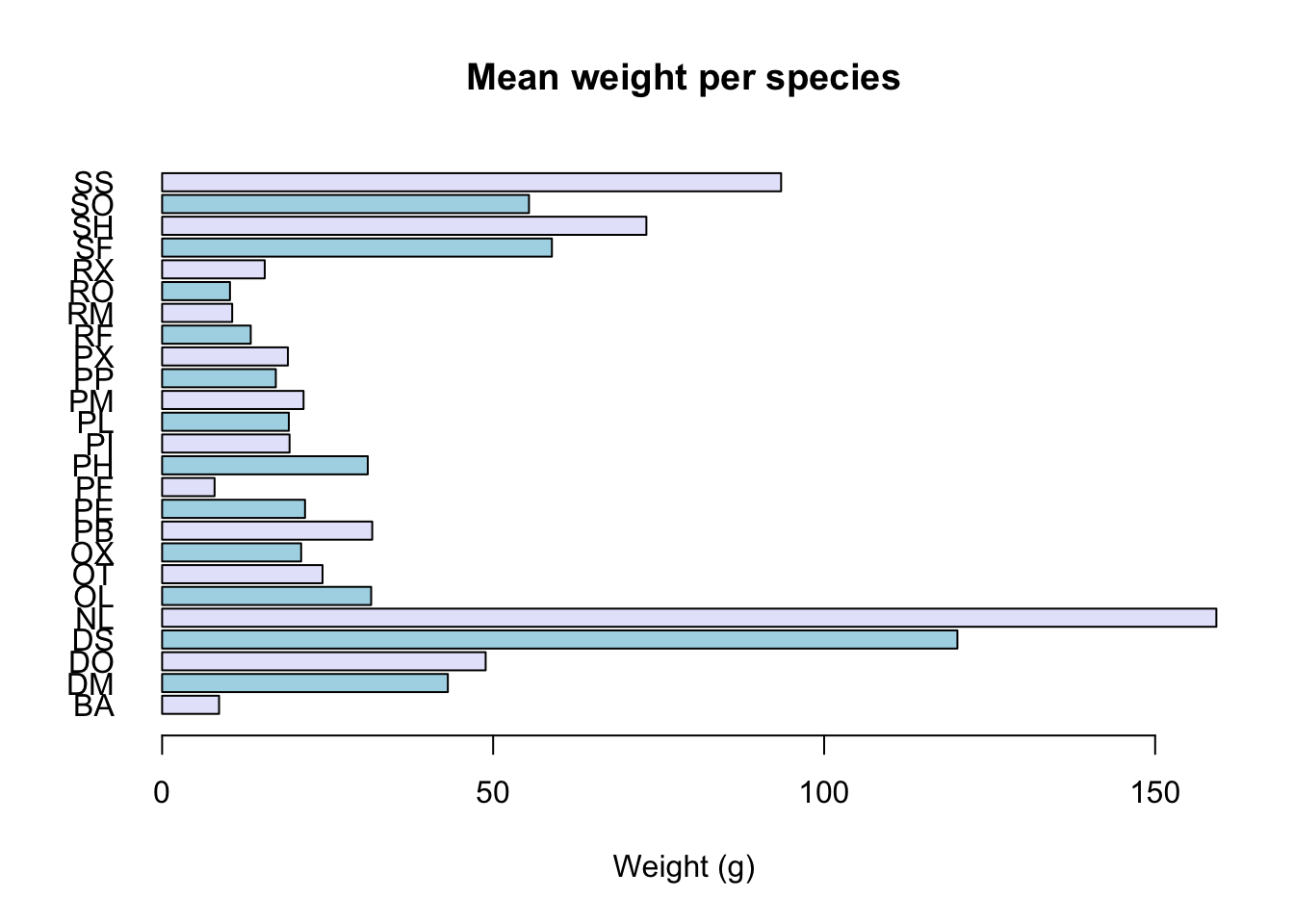## Learning Objectives

• understand how to deal with missing data
• being able to generate summary statistics from the data
• calculate basic statistics across a levels of a factor
• generate a plot from the summary statistics
• write out a data frame as CSV

# Calculating statistics

Let’s get a closer look at our data. For instance, we might want to know how many animals we trapped in each plot, or how many of each species were caught.

To get a vector of all the species, we are going to use the unique() function that tells us the unique values in a given vector:

unique(surveys$species) The function table(), tells us how many of each species we have: table(surveys$species)

We can create a table with more than one field. Let’s see how many of each species were captured in each plot:

table(surveys$plot, surveys$species)

R has a lot of built in statistical functions, like mean(), median(), max(), min(). Let’s start by calculating the average weight of all the animals using the function mean():

mean(surveys$wgt) ##  NA Hmm, we just get NA. That’s because we don’t have the weight for every animal and missing data is recorded as NA. By default, all R functions operating on a vector that contains missing data will return NA. It’s a way to make sure that users know they have missing data, and make a conscious decision on how to deal with it. When dealing with simple statistics like the mean, the easiest way to ignore NA (the missing data) is to use na.rm=TRUE (rm stands for remove): mean(surveys$wgt, na.rm=TRUE)
##  42.67

In some cases, it might be useful to remove the missing data from the vector. For this purpose, R comes with the function na.omit:

wgt_noNA <- na.omit(surveys$wgt) # Note: this is shorthand for wgt_noNA <- surveys$wgt[!is.na(surveys$wgt)] For some applications, it’s useful to keep all observations, for others, it might be best to remove all observations that contain missing data. The function complete.cases() removes any rows that contain at least one missing observation: surveys_complete <- surveys[complete.cases(surveys), ] # remove rows with any missing values After we remove rows with missing values, we need to redo the factors. This is because R “remembers” all species that were found in the original dataset, even though we have now removed them. str(surveys$species)           # factors in the original surveys$species str(surveys_complete$species)  # factors in the subset surveys_complete$species (many are not actually present in the column anymore) surveys_complete$species <- factor(surveys_complete$species) # redo factors on this subset str(surveys_complete$species)  # factors in the subset surveys_complete$species after refactoring ### Challenge 1. To determine the number of elements found in a vector, we can use use the function length() (e.g., length(surveys$wgt)). Using length(), how many animals have not had their weights recorded?

2. What is the median weight for the males?

3. What is the range (minimum and maximum) weight?

4. Bonus question: what is the standard error for the weight? (hints: there is no built-in function to compute standard errors, and the function for the square root is sqrt()).

# Statistics across factor levels

What if we want the maximum weight for all animals, or the average for each plot?

R comes with convenient functions to do this kind of operation: functions in the apply family.

For instance, tapply() allows us to repeat a function across each level of a factor. The format is:

tapply(columns_to_do_the_calculations_on, factor_to_sort_on, function)

If we want to calculate the mean for each species (using the complete dataset):

species_mean <- tapply(surveys_complete$wgt, surveys_complete$species, mean)

### Challenge

1. Create new objects to store: the standard deviation, the maximum and minimum values for the weight of each species
2. How many species do you have these statistics for?
3. Create a new data frame (called surveys_summary) that contains as columns:
• species the 2 letter code for the species names
• mean_wgt the mean weight for each species
• sd_wgt the standard deviation for each species
• min_wgt the minimum weight for each species
• max_wgt the maximum weight for each species

# Creating a barplot

The mathematician Richard Hamming once said, “The purpose of computing is insight, not numbers”, and the best way to develop insight is often to visualize data. Visualization deserves an entire lecture (or course) of its own, but we can explore a few features of R’s base plotting package.

Let’s use the surveys_summary data that we generated and plot it.

R has built in plotting functions.

barplot(surveys_summary$mean_wgt)The axis labels are too big though, so you can’t see them all. Let’s change that. barplot(surveys_summary$mean_wgt, cex.names=0.4)Alternatively, we may want to flip the axes to have more room for the species names:

barplot(surveys_summary$mean_wgt, horiz=TRUE, las=1)Let’s also add some colors, and add a main title, label the axis: barplot(surveys_summary$mean_wgt, horiz=TRUE, las=1,
col=c("lavender", "lightblue"), xlab="Weight (g)",
main="Mean weight per species")### Challenge

1. Create a new plot showing the standard deviation for each species. Choose one or more colors from here. (If you prefer, you can also specify colors using their hexadecimal values #RRGGBB.)

There are lots of different ways to plot things. You can do plot(object) for most classes included in R base. To explore some of the possibilities:

?barplot
?boxplot
?plot.default
example(barplot)

There’s also a plotting package called ggplot2 that adds a lot of functionality. The syntax takes some getting used to but it’s extremely powerful and flexible.

If you wanted to output this plot to a pdf file rather than to the screen, you can specify where you want the plot to go with the pdf() function. If you wanted it to be a JPG, you would use the function jpeg() (other formats available: svg, png, ps).

Be sure to add dev.off() at the end to finalize the file. For pdf(), you can create multiple pages to your file, by generating multiple plots before calling dev.off().

pdf("mean_per_species.pdf")
barplot(surveys_summary\$mean_wgt, horiz=TRUE, las=1,
col=c("lavender", "lightblue"), xlab="Weight (g)",
main="Mean weight per species")
dev.off()

# More analysis options: libraries

In our lessons so far, we’ve used many of R’s built-in functions. These are enough for a lot of data manipulation and basic statistics.

R also has many packages available that provide extra functions. Many of these packages might already be installed on your computer, and others you can easily install. Many packages can be found in the CRAN repository, which you can automatically access from R with the install.packages() function.

For instance, to install the RSQLite package, run

install.packages("RSQLite")

This package provides functions to query an SQLite database, like the portal_mammals.sqlite database we worked with previously. Once the package is installed we can load it with the library() function:

library("RSQLite")
## Loading required package: DBI

Now the functions in this library are available for us to use. We can get some information on the package contents with

library(help="RSQLite")

And as always, we can look up help on individual functions. If you have loaded the library, try help(dbSendQuery).

We can now use the RSQLite functions to try querying the database:

# Open the file (connect to the database)
mammals_db <- dbConnect(SQLite(), "data/portal_mammals.sqlite")
# See what tables are in it
dbListTables(mammals_db)
##  "plots"   "species" "surveys"
# Run an SQL query
queryResult <- dbSendQuery(mammals_db, 'SELECT DISTINCT genus FROM species WHERE taxa="Rodent"')
# Fetch will get the results as a data frame
fetch(queryResult, n=10)
##              genus
## 1          Baiomys
## 2        Dipodomys
## 3          Neotoma
## 4        Onychomys
## 5      Chaetodipus
## 6       Peromyscus
## 7      Perognathus
## 8  Reithrodontomys
## 9         Sigmodon
## 10          Rodent
# Close the file (disconnect from the database)
dbDisconnect(mammals_db)
##  TRUE

Previous: Manipulating data Next: Getting help